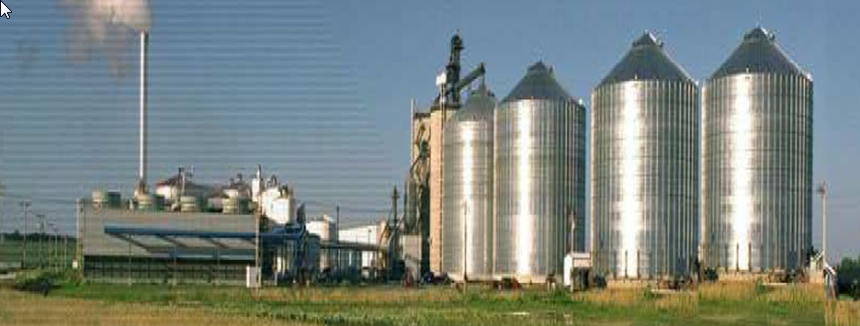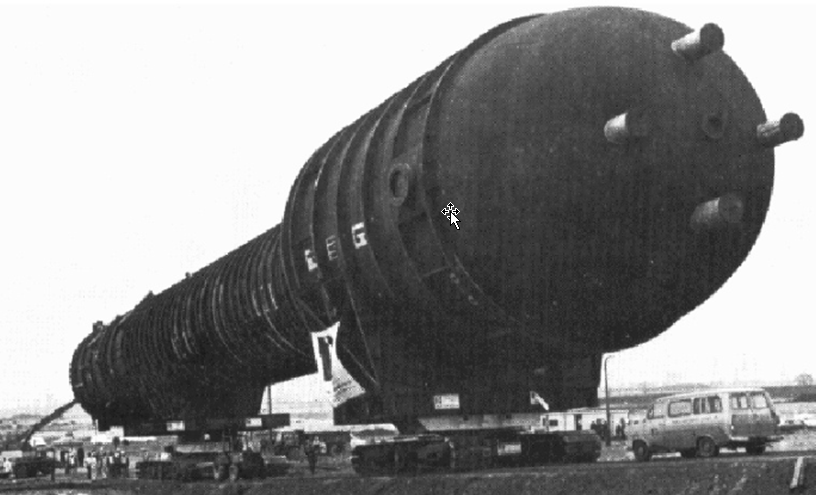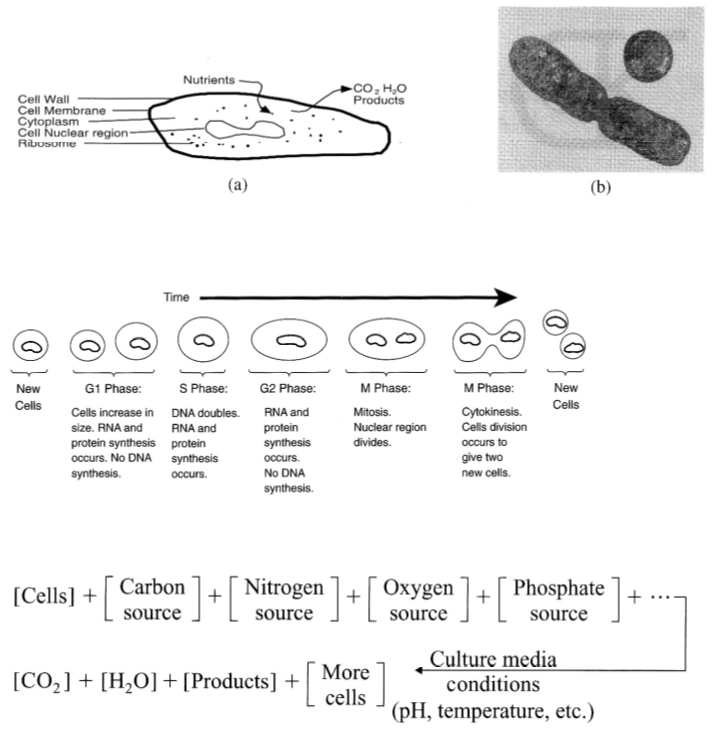# Bioreactors¶

Why the dinosaurs died out is not known, but it is supposed to be because they had minute brains and devoted themselves to the growth of weapons of offense in the shape of numerous horns.

—Bertrand Russell

We have examined a number of conservation and rate equations that are applicable to all Chemical and Biological Engineering processes. However, it is useful to examine some processes specifically biological in nature.

We could cover biological heat transfer, biological mass transfer, biological reactors, etc.

Here we will focus on biological reactors, i.e., bioreactors.

## What is a bioreactor¶

An apparatus for growing organisms (yeast, bacteria, or animal cells) under controlled conditions. Used in industrial processes to produce pharmaceuticals, vaccines, or antibodies. Also used to convert raw materials into useful byproducts such as in the bioconversion of corn into ethanol.Glacial Lakes Energy in Watertown, South Dakota

57+ million gallons per year ethanol productionWorld’s largest industrial fermenter (as of 1978)

The fermenter is 200 feet high and 25 feet in diameter

## Bioreactor basics¶

• They are systems or devices that supports a biologically active environment

• They are vessels in which a chemical process is carried out which involves organisms or biochemically active substances derived from such organisms

• They can be either aerobic or anaerobic

• They are commonly cylindrical, ranging in size from liters to cubic meters, and are often made of stainless steel

• They supply a homogeneous (same throughout) environment by constantly stirring the contents.

• They give the cells a controlled environment by ensuring the same temperature, pH, and oxygen levels.

## Important biological issues¶Limiting substrate: The substrate or substrate(s) (energy source, carbon source and/or nutrient source) which is/are first exhausted in batch growth. This substrate(s) has a direct influence on the kinetics of cell growth.

In excess: The substrate or substrate(s) (energy source, carbon source and/or nutrient source) which is/are not exhausted at the end of batch growth. This substrate(s) has no effect on the kinetics of cell growth.

Exercise: Cell numbers versus time in a bioreactor

Considering the above factors, make a sketch of the number of cells over time in a bioreactor.

## Quantifying reactions rates for enzymes¶

Maud Menten and Leonor MichaelisMichaelis-Menten kinetics

$v_{0} = r_{P} = - r_{S} = \frac{ V_{\max } \left[ S \right]} {K_{m} + \left[ S \right]}$

where, $$v_{0}$$ is the initial ‘reaction velocity’, $$r_{p}$$ is the rate of production of the product, $$r_{s}$$ is the rate of production of the substrate, $$S$$ is substrate, $$P$$ is product, and $$[]$$ represents concentration.

This equation governs the initial reaction rates (i.e., not including the reversion of products).

Exercise: Michaelis-Menten kinetics

Consider the Michaelis-Menten equation.

• What are the units of $$V_{\max}$$ and $$K_{m}$$?

• Sketch out the rate of product formation as a function of substrate concentration.

• What is the order of the reaction at ‘relatively low’ and ‘relatively high’ substrate concentrations?

## Reactor types¶

Chemostat (chemical environment is static)

A bioreactor to which fresh medium is continuously added, while culture liquid is continuously removed to keep the culture volume constantWhat type of reactor is this?

Exercise: Chemostate analysis

Consider this schematic:where

• $$[S]$$ is the concentration of growth limiting substrate

• $$F$$ or $$\dot V$$ is the volumetric flow rate of $$S$$ into the chemostat

• $$V$$ is the reactor volume

• $$x$$ is the biomass per unit volume (x = biomass/V)

• $$\mu$$ is the biomass growth rate

• $$\tau$$ is the residence time in the reactor ($$\tau = V/F$$)

• D is the dilution rate ($$D = F/V$$)

• Cell growth kinetics: $$r_{x} = \mu \, x$$

Questions:

1. What are the units for $$r_{x}$$?

2. Assuming sterile feed, write a steady-state material balance for $$x$$.

3. Make a plot of $$x$$ (y-axis) and $$S$$ (second y-axis) as a function of $$D$$ (x-axis).

4. What happens if $$D$$ is very large?

## Considerations in bioreactor design¶

• Oxygenation for aerobic microorganisms

• Temperature management

• Methods for monitoring the culture

• SterilityExercise: Bioreactor features

Make a list of the features that make a bioreactor different from a standard chemical reactor.

Sketch a bioreactor and include all of the equipment that you think is necessary.